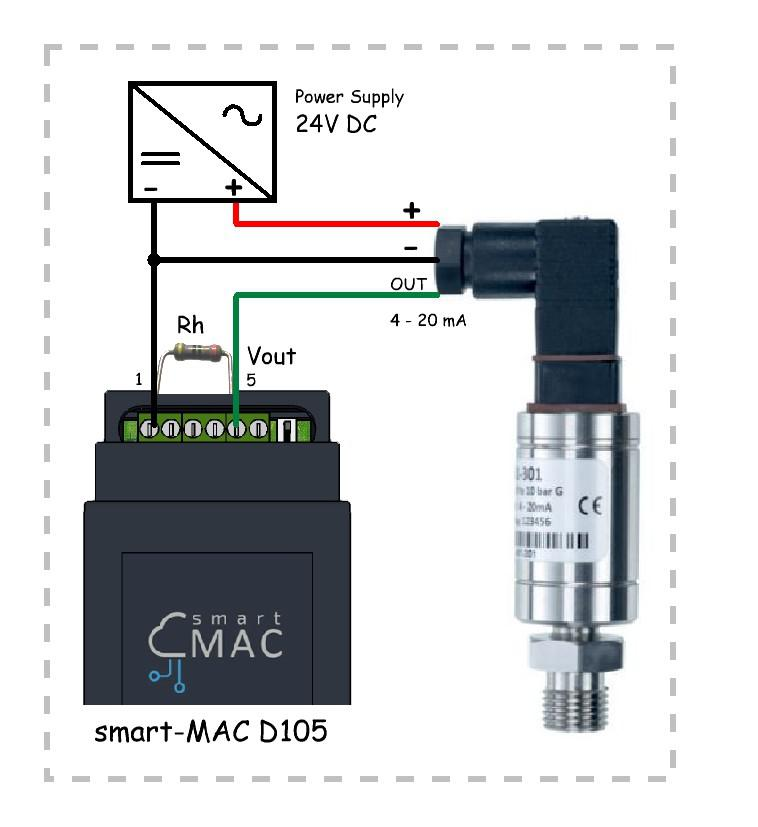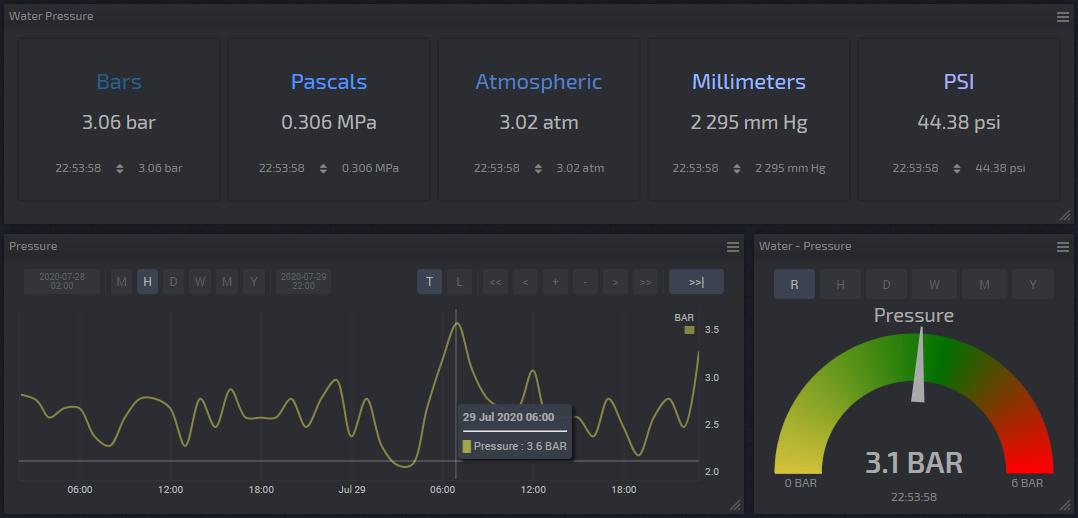# D105: Connecting the sensor with a 4-20mA current loop

### 4-20mA Current loop sensor

Industrial sensors often use a current signal to transmit data. The current loop transmitter does not use a voltage source, but a current source, which allows the signal to be transmitted over long distances. The sensors themselves require a separate 9-30V power supply.

A sensor with a 4-20mA current loop can be connected to the smart-MAC D105 universal meter according to a simple circuit with
using calibrated resistance Rh. As a result, we obtain the voltage Vout at the output of the sensor, which is easily measured at the analog input D105, terminal 5 (ADC), in the range 0 - 15V.Wiring diagram of the sensor with a current loop 4-20mA to smart-MAC D105

The resistance Rh should be selected according to the table based on the required voltage at the output Vout, the supply voltage of the current sensor and the voltage of the available external power supply (Power Supply).

 Vout Output Voltage Range 1-5 V 2-10 V Required load resistance Rh 240 Om 510 Оm Vout at 4 mA (minimum sensor value) 0.96 V 2.04 V Vout at 20 mA (maximum sensor value) 4.8V 10.2 V Recommended Power Supply Voltage 15 V 24 V
Selection table for resistance Rh according to the output voltage Vout

#### The selection of resistance Rh and the formula for converting Vout into a measured quantity.

The voltage Vout, at the analog input of the D105 device, depends on the resistance Rh and is calculated according to Ohm's law, where I is the current on the sensor within 4-20mA.

```Vout = I * Rh
```

Minimum voltage Vout at 4mA:

` Vout min = 0.004 * Rh`

Maximum voltage Vout at a current of 20mA:

` Vout max = 0.02 * Rh`
##### Consider the example of connecting a pressure sensor 0-400 bar.

1. With a resistance of Rh 500 Ohm, the voltage Vout will change in the range of 2-10V:

```Vout min = 0.004 * 510 = 2.04V
Vout max = 0.02 * 510 = 10.2V```

At the same time, the voltage will drop by an external power source by Vout, a maximum of 10V. For the correct selection of an external power source, it is necessary to add a maximum voltage drop of 10V to the minimum sensor power supply, for example, 9V. So the voltage on the external power source should be at least 19V (24V recommended).

Next, we determine how many Bar of pressure on the sensor falls on each 1V of voltage Vout, knowing the possible pressure range of 0-400 bar and the range of variation of voltage Vout of 2.4 - 10.2V

`400 bar / ( Vout max - Vout min ) = 400/(10.2-2.04) = 49.02`

Let us make a formula for the linear dependence of f (x) between the pressure at the sensor and the voltage Vout.

`Pressure [Bar] = (Vout - 2.04) * 49.02`

2. With a resistance of Rh 250 Ohms, the voltage Vout will vary in the range of 1-5V for pressure of 0-400 bar.
The maximum voltage drop at an external power source will be 5V, so you need to select an external power source with a voltage of at least 14V (9 + 5). For every 1V, there will be 100 bar and the linear dependence formula f (x) will take the form:

```400 Bar/ ( Vout max - Vout min ) = 400/(5-1) = 100 Bar for every 1V
Pressure [Bar] = (Vout - 1) * 100```

As you can see, the option with a resistance of Rh 500 Ohms will provide a smaller measurement step and more accurate results, but will require an external power source with a higher voltage of 19V (24V recommended).

#### Dashboard widget settings.

Add a widget indicator, chart or table to the board.

Select your smart-MAC D105 device, in the "Arithmetic Operation" section, select the function f (x) and write down the function to calculate the pressure in the units of measure you need.

##### For example, for a voltage Vout within 2-10V with a resistance of Rh 500 Ohms.

Bars [bar]

`d.ADC>2.04 ? (d.ADC - 2.04) * 49.02 : 0`

Pascals [MPa]

`d.ADC>2.04 ? (d.ADC - 2.04) * 49.02 * 0.1 : 0`

Atmospheres [atm]

`d.ADC>2.04 ? (d.ADC - 2.04) * 49.02 * 0.986923 : 0`

Millimeters of mercury [mmHg Art.]

`d.ADC>2.04 ? (d.ADC - 2.04) * 49.02 * 750.06 : 0`

Pound per square inch [psi]

`d.ADC>2.04 ? (d.ADC - 2.04) * 49.02 * 14.5038 : 0`

#### Set up notifications on the device and control the built-in relay.

To configure notifications on the D105 device and automatically control the built-in relay according to the pressure level, it is necessary to determine the formula for calculating the voltage at the analog input Vout at the corresponding pressure level on the Bar sensor.

`Vout = (Bar+2.04*49.02)/49.02 = (Bar + 100) / 49.02`

Where:
Bar - the pressure level in bars, at which an alert should come or a relay is activated;
Vout - the voltage at the analog input, at the appropriate pressure.